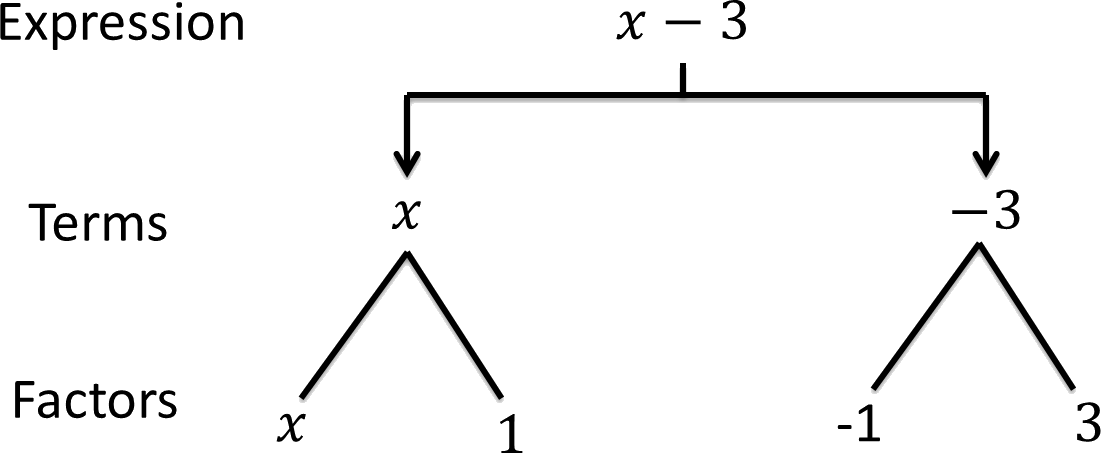1. /
2. CBSE
3. /
4. Class 07
5. /
6. Algebraic Expressions worksheet for...

# Algebraic Expressions worksheet for class 7### myCBSEguide App

Download the app to get CBSE Sample Papers 2023-24, NCERT Solutions (Revised), Most Important Questions, Previous Year Question Bank, Mock Tests, and Detailed Notes.

## Algebraic Expressions worksheet for class 7 Important Topics

• Introduction: Formation of an equation
• Terms of an expression : Factors and coefficients of a term
• Like and unlike terms : Monomials, Binomials, Trinomials and Polynomials
• Addition and subtraction of algebraic expressions
• Finding the value of an expression
• Using algebraic expression – formulas and rules [Perimeter formula; area formula]
• Simple linear equations
• Patterns in numbers and geometry## Some important Facts about Algebraic Expressions worksheet for class 7

1. Algebraic expressions are formed from variables and constants. We use the operations of addition, subtraction, multiplicationand division on the variables and constants to form expressions. For example, the expression 4xy + 7 is formed from the variables x and y and constants 4 and 7. The constant 4 and the variables x and y are multiplied to give the product 4xy and the constant 7 is added to this product to give the expression.
2. Expressions are made up of terms. Terms are added to make an expression. For example, the addition of the terms 4xy and 7 gives the expression 4xy + 7.
3. A term is a product of factors. The term 4xy in the expression 4xy + 7 is a product of factors x, y and 4. Factors containing variables are said to be algebraic factors.
4. The coefficient is the numerical factor in the term. Sometimes anyone factor in a term is called the coefficient of the remaining part of the term.
5. Any expression with one or more terms is called a polynomial. Specifically a one term expression is called a monomial; a two-term exp

## NCERT class 7Mathematics Solved Worksheets

• Chapter 1 – Integers
• Chapter 2 – Fractions and Decimals
• Chapter 3 – Data Handling
• Chapter 4 – Simple Equations
• Chapter 5 – Lines and Angles
• Chapter 6 – Practical Geometry
• Chapter 7 – The Triangle and its Properties
• Chapter 8 – Congruence of triangles
• Chapter 9 – Comparing Quantities
• Chapter 10 – Rational numbers
• Chapter 11 – Perimeter and Area
• Chapter 12 – Algebraic Expressions
• Chapter 13 – Exponents and Powers
• Chapter 14 – Symmetry
• Chapter 15 – Visualizing Solid Shapes

## FINDING THE VALUE OF AN EXPRESSION

We know that the value of an algebraic expression depends on the values of the variables forming the expression. There are a number of situations in which we need to find the value of an expression, such as when we wish to check whether a particular value of a variable satisfies a given equation or not. We find values of expressions, also, when we use formulas from geometry and from everyday mathematics. For example, the area of a square is l 2 , where l is the length of a side of the square. If l = 5 cm., the area is 52 cm2 or 25 cm2 ; if the side is 10 cm, the area is 102 cm2 or 100 cm2 and so on. We shall see more such examples in the next section.

To download Printable worksheets for class 7 Mathematics and Science; do check myCBSEguide app or website. myCBSEguide provides sample papers with solution, test papers for chapter-wise practice, NCERT solutions, NCERT Exemplar solutions, quick revision notes for ready reference, CBSE guess papers and CBSE important question papers. Sample Paper all are made available through the best app for CBSE students and myCBSEguide website.### Test Generator

Create question paper PDF and online tests with your own name & logo in minutes.### myCBSEguide

Question Bank, Mock Tests, Exam Papers, NCERT Solutions, Sample Papers, Notes